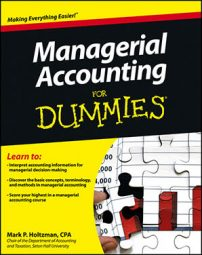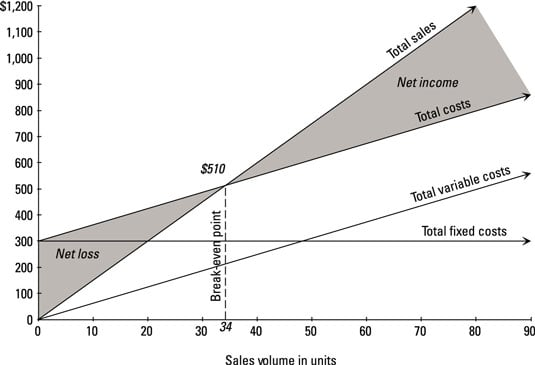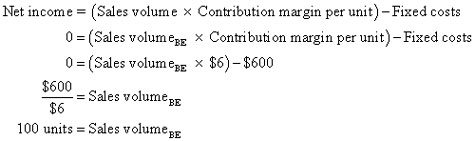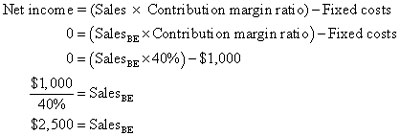##### Managerial Accounting For DummiesHow much do you need to sell in order to break even? The break-even point (BE) is the amount of sales needed to earn zero profit — enough sales so that you don’t earn a loss, but insufficient sales to earn a profit. You can use a couple of different ways — graphs and formulas — to analyze where your break-even point falls.

## Draw a graph to find the break-even point

In a cost-volume-profit graph, the break-even point is the sales volume where the total sales line intersects with the total costs line. This sales volume is the point at which total sales equals total costs.

Suppose that, as with the basketball example earlier in the chapter, a company sells its products for \$15 each, with variable costs of \$6 per unit and total fixed costs of \$300. The graph indicates that the company’s break-even point occurs when the company sells 34 units.For many products (like basketballs) you can only sell whole units. Therefore, if you sell whole units, the break-even point must always be a whole number. This fact means that if break-even analysis results in some fractional volume of sales (such as 33.33333 units), you should always round up (in this case, to 34 units), even if the fraction is closer to the lower whole number than the higher number.

If your break-even point equals 33.0001, round it up to 34. If you rounded down (to 33 units), then the actual sales volume would be below the break-even point, and at this volume level, your company would report a net loss.

## Employ the formula approach to find the break-even point

When you need to solve actual problems, drawing graphs isn’t always practical. In that case, you want to rely on the three formulas that use contribution margin to measure net income.

The break-even point is where net income is zero, so just set net income equal to zero, plug whatever given information you have into one of the equations, and then solve for sales or sales volume. Better yet: At the break-even point, total contribution margin equals fixed costs.

Suppose a company has \$30,000 in fixed costs. How much total contribution margin does it have to generate in order to break even?

Net income = Total contribution margin – Fixed costs
0 = Total contribution marginBE – Fixed costs
0 = Total contribution marginBE – \$30,000
\$30,000 = Total contribution marginBE

Here, too, at the break-even point, total contribution margin equals fixed costs of \$30,000. Now suppose a company has contribution margin per unit of \$6 and fixed costs of \$600. What’s the break-even point in units?Another company has a contribution margin ratio of 40 percent and fixed costs of \$1,000. Sales price is \$1 per unit. What’s the break-even point in dollars?You can express break-even point in units or dollars. If your formula gives you units and you want dollars, multiply the number of units by the sales price. If your formula gives you dollars and you want units, just divide by the sales price.